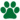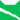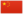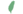Millie

《风暴来袭》第十一章 (Q3766)独行猫 (Q648)雷族 (Q627)雷族 猫后雷族 武士雷族 长老米莉 Millie  蜜妮

Q2934：雷族母猫，原宠物猫，自《预视力量》登场的角色

## 细节

### 外貌

• 她是一只身形娇小、:猫物表蓝色眼睛、:猫物表浅灰色虎斑的短毛母猫，:143一只耳朵撕裂。:268
• 她生前近乎失聪。:2章

## 登场书目

• 预视力量 (Q152)[配角][首次登场🐱]
• 暗河汹涌 (Q153)[配角]
• 驱逐之战 (Q154)[配角]
• 天蚀遮月 (Q155)[配角]
• 暗夜长影 (Q156)[配角]
• 拂晓之光 (Q157)[配角]
• 第四学徒 (Q158)[配角]
• 战声渐近 (Q159)[配角]
• 暗夜密语 (Q160)[配角]
• 月光印记 (Q161)[配角]
• 武士归来 (Q162)[配角]
• 群星之战 (Q163)[配角]
• 学徒探索 (Q170)[配角]
• 雷影交加 (Q171)[配角]
• 天空破碎 (Q172)[配角]
• 极夜无光 (Q173)[配角]
• 烈焰焚河 (Q174)[配角]
• 风暴来袭 (Q175)[配角]
• 迷雾明光 (Q4621)[配角]
• 黑莓星的风暴 (Q182)[配角]
• 虎心的阴影 (Q185)[猫物闲角]
• 鸦羽的拷问 (Q186)[猫物闲角]
• 松鼠飞的希冀 (Q3561)[配角]
• 灰条的誓言 (Q4057)[仅于幻象][死亡时刻✝]
• 冬青叶的故事 (Q187)[配角]
• 雾星的征兆 (Q188)[猫物闲角]
• 鸽翅的沉默 (Q192)[配角]
• 褐皮的族群 (Q3566)[猫物闲角]
• 斑毛的反抗 (Q4337)[配角]
• 武士失踪 (Q207)[主要角色][视角人物][首次登场🐱]
• 武士避难 (Q208)[主要角色]
• 武士回归 (Q209)[主要角色]
• 族群的猫 (Q200)[配角]
• 终极指南 (Q204)[配角]

## 参考文献

这篇文章基于CC BY-SA 3.0许可使用了猫武士维基（英语）Millie一文中的部分内容。